# Circles

### From DT Online

##### Description

Circles are very familiar but have associated with them terminology, properties and characteristics with which all involved with design and technology should understand.

A particular property of a circle is that its radius can be stepped off around its circumference exactly 6 times. In effect, this constructs 6 equi-lateral triangles, the interior angles of which are all 600, and the sum of all angles at the centre of any polygon must equal 3600.

The sides of these equi-lateral triangles become chords of the circle and should not be confused with the length of an arc, which is longer. Lengths of arcs can be found by dividing the circumference by the number of sides. The circumference is calculated as 2πr or πD (π is a constant obtained by dividing circumference by diameter - i.e. approximately 3.142)".

##### Constructions
 Angle in a Semi-Circle Any angle drawn by connecting each end of a semi-circle diameter to point on its circumference is a right angle. Note: If angles are drawn in each half of the circle the result would be a particular case of cyclic quadlitaleral which makes it easier to see the more general case that the sum of opposite internal angles of any cyclic quadlitaleral is always equal to 1800. ``` ```Bisecting Chords The perpendicular bisectors of any two chords to a circle will intersect at the centre of that circle In practice, a 3rd chord is also bisected as shown to serve as an accuracy check when finding the circle centre.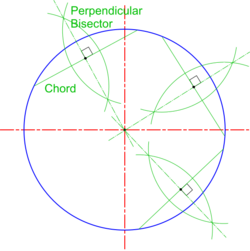Intersecting Chords Theorem Looking at one of the chords and its bisector above, it can be proved that A x B = C x D. It can be derived from this that finding the centre of an arch doorway or window for example can be found by the formula: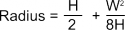Where: W is the length of the chord defining the base of the arc and H is the height measured at the midpoint of the arc's base. Note: This is a particular case of the Intersecting Chords Theorem which states that the relationship (A x B = C x D) is true for any two intersecting chords, whether or not one of them is a diameterTangent from a Point Outside a Circle For most practical purposes, especially with large-scale work, it can be satisfactory simply to draw a line touching a circle as the tangent There may be occasions however when it is important to know exactly where the tangent touches the circle circumference (i.e. the Point of Tangency) For points on the circumference, the tangent is simply drawn as a line at right angles to a line joining the point to the circle centre (i.e. the Normal). Points outside the circumference exploit the property of Angles in a Semi-Circle as described above. For points outside the circle, join the point to the circle centre and construct a semi-circle on this line. Where the semi-circle cuts the circumference provides the Point of Tangency to which may be drawn both the Normal and Tangent required. Note: This concept is the basis of the design of Centre Squares ( or Centre Finders)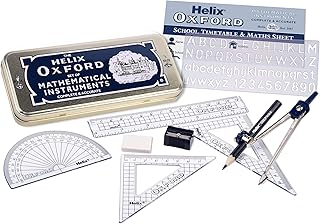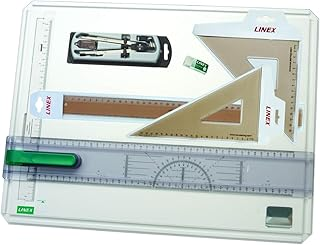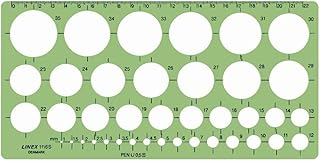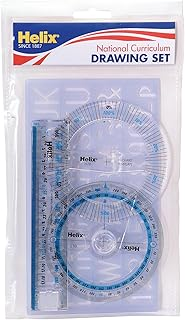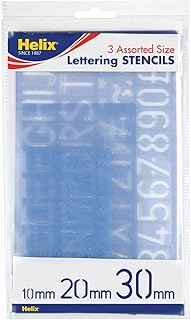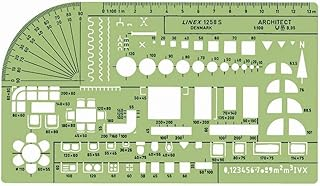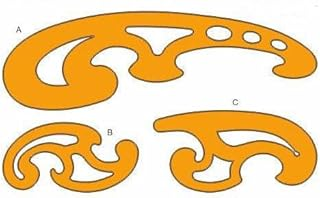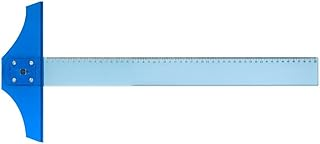Helix Oxford Maths SetLinex Student Saver BundleHelix Precision Plus Drawing SetPrimary Shape Template SetLinex Circle TemplateHelix National Curriculum Drawing SetHelix 3 Piece Stencil SetLinex Universal Architects TemplateFrench Curve Transparent TemplateT-Square